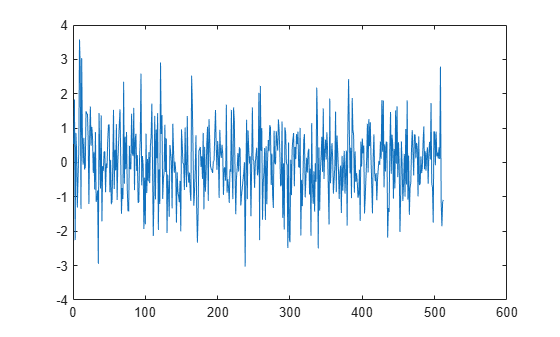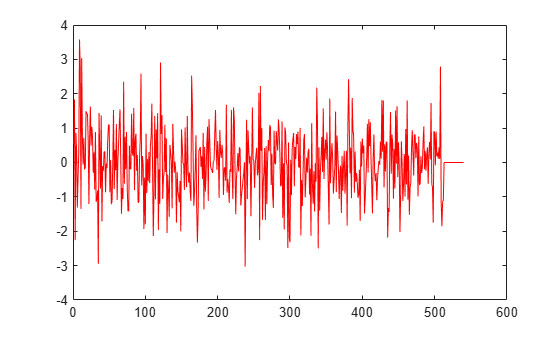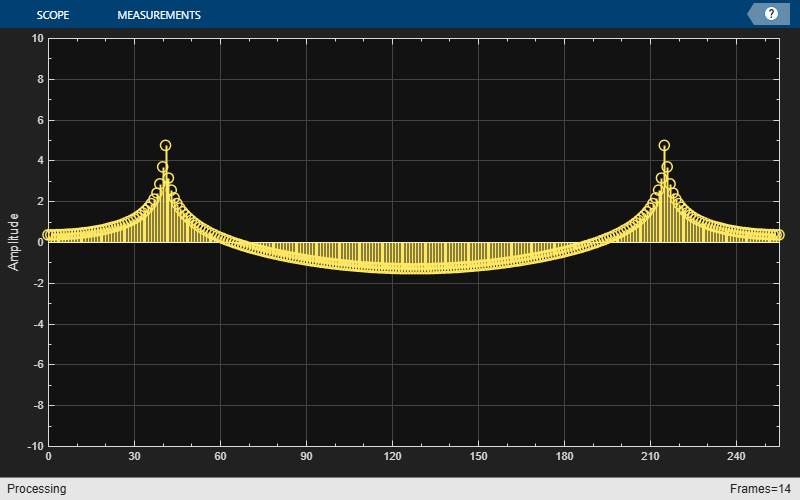dsp.AsyncBuffer

Description

The dsp.AsyncBuffer System object™ writes samples to and reads samples from a first-in, first-out (FIFO) buffer. The write method writes data to the buffer, and the read method reads data from the buffer. When creating the object, you can set the number of samples (rows) of the buffer using the Capacity property. The number of channels (columns) is set during the first call to write. Initialize the buffer by calling write or setup before the first call to read.

The data that you write occupies the next available space in the buffer. If the buffer is full and all the data within it is unread (asyncBuff.NumUnreadSamples == asyncBuff.Capacity), the object overwrites the oldest data with any new data that comes in. The buffer removes data only when the data is overwritten, so you can reread data from the past. The dsp.AsyncBuffer object supports writing and reading variable frame size signals. For examples, see Read Variable Frame Sizes from Buffer and Write Variable Frame Sizes to Buffer.

To write and read samples from a FIFO buffer:

• Create a dsp.AsyncBuffer object and set the properties of the object.

• Call write to write samples to the buffer.

• Call peek to read samples without changing the number of unread samples in the buffer.

Creation

Description

example

asyncBuff = dsp.AsyncBuffer returns an async buffer System object, asyncBuff, using the default properties.

asyncBuff = dsp.AsyncBuffer(cap) sets the Capacity property to cap.

Example: asyncBuff = dsp.AsyncBuffer(200000);

Properties

expand all

Unless otherwise indicated, properties are nontunable, which means you cannot change their values after calling the object. Objects lock when you call them, and the release function unlocks them.

If a property is tunable, you can change its value at any time.

Number of writable/readable rows in the buffer, specified as a positive integer greater than or equal to 2. The number of rows during each write to the buffer must not exceed the capacity of the buffer. If the buffer is full and all the data within is unread, the object overwrites the oldest data with any new data that comes in. The CumulativeOverrun property returned by info gives the number of samples overrun per channel since the last call to reset. The number of samples overrun is the number of unread samples overwritten.

By default, this property has data type int32.

Example: asyncBuff = dsp.AsyncBuffer(200000);

Data Types: single | double | int8 | int16 | int32 | int64 | uint8 | uint16 | uint32 | uint64

Number of unread samples in each channel (column) of the buffer, specified as an integer greater than or equal to 0. The total number of unread samples in the buffer is NumUnreadSamples × numChann. The variable numChann is the number of channels in the buffer. The number of channels in the buffer is the number of data columns in the first call to write.

The CumulativeUnderrun property returned by the info method gives the number of samples underrun per channel since the last call to reset. Underrun occurs if you attempt to read more samples than available.

Example: asyncBuff = dsp.AsyncBuffer; input = randn(512,1); numUnreadSamples = write(asyncBuff,input)

Data Types: int32

Usage

To write and read samples from the async buffer:

• Create a dsp.AsyncBuffer object and set the properties of the object.

• Call write to write samples to the buffer.

• Call peek to read samples without changing the number of unread samples in the buffer.

Object Functions

To use an object function, specify the System object as the first input argument. For example, to release system resources of a System object named obj, use this syntax:

release(obj)

expand all

 info Get cumulative overrun and underrun read Read data from buffer write Write data to buffer peek Read data from buffer without changing number of unread samples
 step Run System object algorithm release Release resources and allow changes to System object property values and input characteristics reset Reset internal states of System object

Examples

collapse all

The dsp.AsyncBuffer System object™ supports reading variable frame sizes from the buffer.

Create a dsp.AsyncBuffer System object. The input is white Gaussian noise with a mean of 0, a standard deviation of 1, and a frame size of 512 samples. Write the input to the buffer using the write method.

asyncBuff = dsp.AsyncBuffer;
input = randn(512,1);
write(asyncBuff,input);
plot(input)
hold onStore the data that is read from the buffer in outTotal.

Plot the input signal and data that is read from the buffer in the same plot. Read data from the buffer until all samples are read. In each iteration of the loop, randi determines the number of samples to read. Therefore, the signal is read in as a variable-size signal. The prevIndex variable keeps track of the previous index value that contains the data.

outTotal = zeros(size(input));
prevIndex = 0;
end
plot(outTotal,'r')
hold offVerify that the input data and the data read from the buffer (excluding the underrun samples, if any) are the same. The cumulative number of overrun and underrun samples in the buffer is determined by the info function.

S = info(asyncBuff)
S = struct with fields:
CumulativeOverrun: 0
CumulativeUnderrun: 28

The CumulativeUnderrun field shows the number of samples underrun per channel. Underrun occurs if you attempt to read more samples than available.

Write a sine wave of variable frame size to the buffer. Compute the FFT of the sine wave and visualize the result on an array plot.

Initialize the dsp.AsyncBuffer, dsp.ArrayPlot, and dsp.FFT System objects.

asynBuff = dsp.AsyncBuffer;
plotter = dsp.ArrayPlot;
fftObj = dsp.FFT('FFTLengthSource','Property','FFTLength',256);

The sine wave is generated using the sin function in MATLAB. The start and finish variables mark the start and finish indices of each frame. If enough data is cached, read from the buffer and perform the FFT. View the FFT on an array plot.

start = 1;

for Iter = 1 : 2000
numToWrite = randi([200,800]);
finish = start + numToWrite;

inputData = sin(start:finish)';
start = finish + 1;

write(asynBuff,inputData);
X = abs(fftObj(x));
plotter(log(X));
end
endRead data from the async buffer without changing the number of unread samples using the peek function.

Create a dsp.AsyncBuffer System object™. The input is a column vector of 100 samples, 1 to 100. Write the data to the buffer.

asyncBuff = dsp.AsyncBuffer
asyncBuff =
AsyncBuffer with properties:

Capacity: 192000

input = (1:100)';
write(asyncBuff,input);

Peek at the first three samples. The output is [1 2 3]'.

out1  = peek(asyncBuff,3)
out1 = 3×1

1
2
3

The NumUnreadSamples is 100, indicating that the peek function has not changed the number of unread samples in the buffer.

ans = int32
100

After peeking, read 50 samples using the read function. The output is [1:50]'.

out2 = 50×1

1
2
3
4
5
6
7
8
9
10
⋮

The NumUnreadSamples is 50, indicating that the read function has changed the number of unread samples in the buffer.

ans = int32
50

Now peek again at the first three samples. The output is [51 52 53]'. Verify that the NumUnreadSamples is still 50.

out3  = peek(asyncBuff,3)
out3 = 3×1

51
52
53

ans = int32
50

Read 50 samples again. The output now contains the sequence [51:100]'. Verify that NumUnreadSamples is 0.

out4 = 50×1

51
52
53
54
55
56
57
58
59
60
⋮

ans = int32
0

Limitations

Before calling the read method, you must initialize the buffer by calling either the write or setup method. For an example, see Why Does the dsp.AsyncBuffer Object Error When You Call read Before write?

Extended Capabilities

Blocks

Introduced in R2017a

DSP System Toolbox DocumentationGet trial now